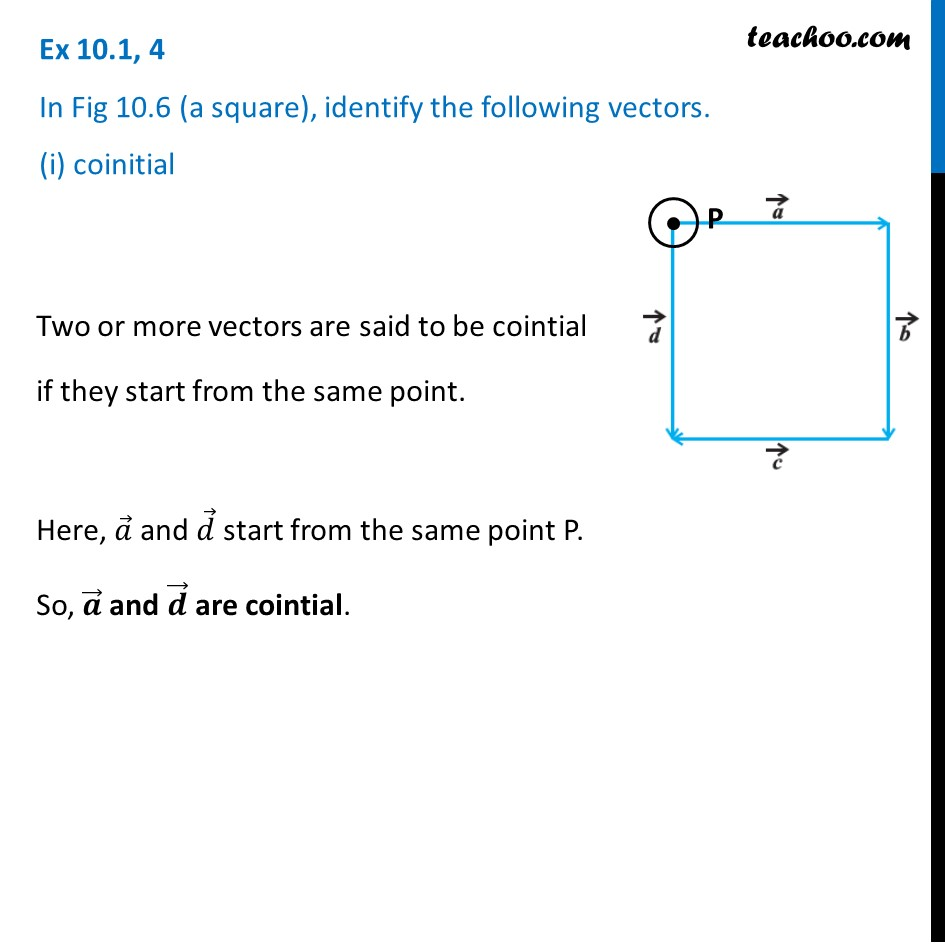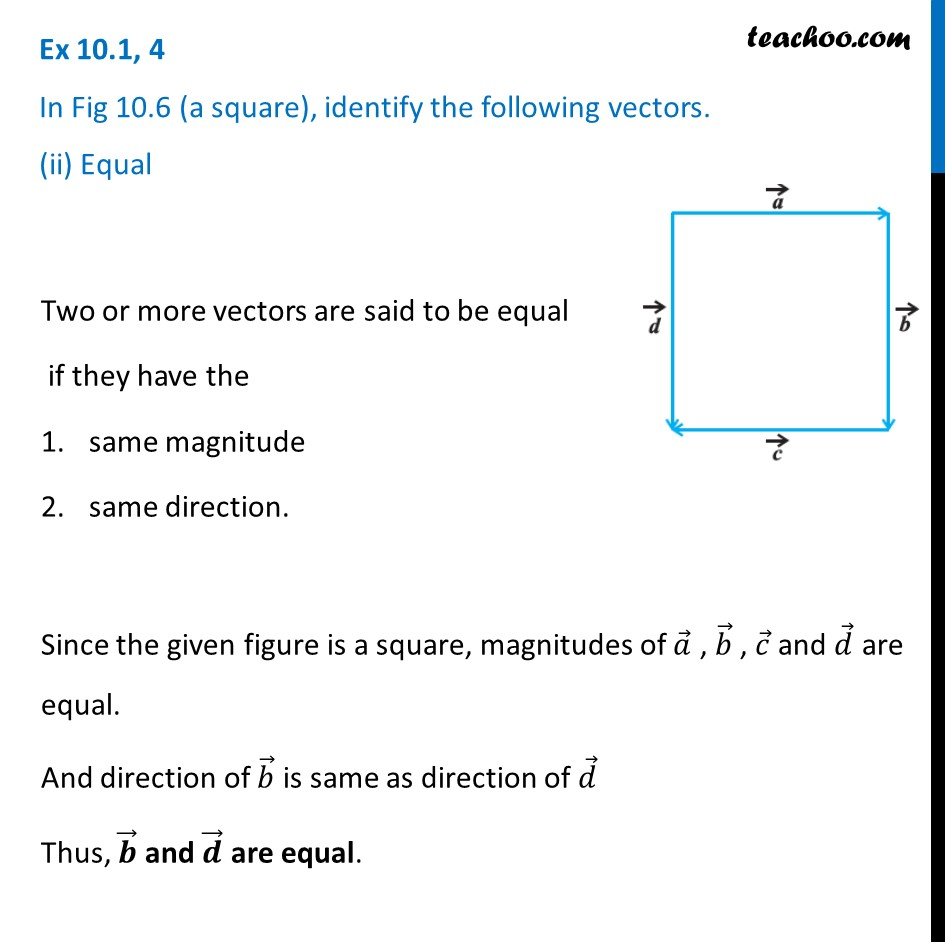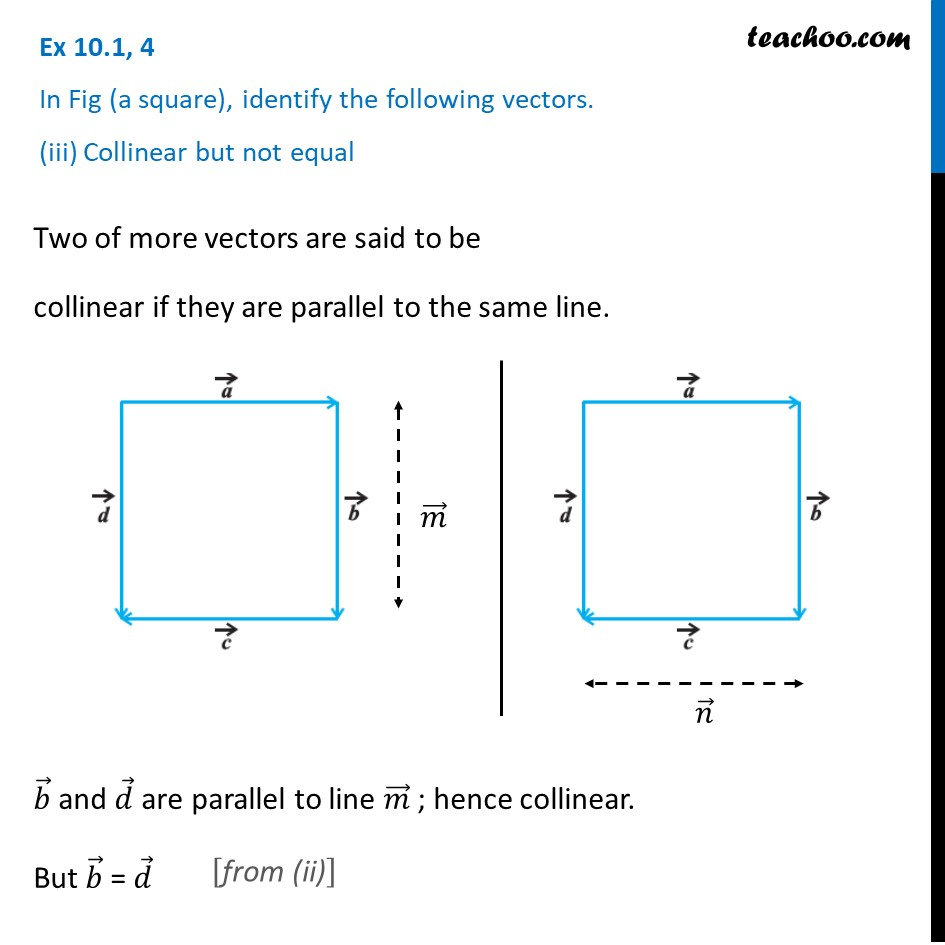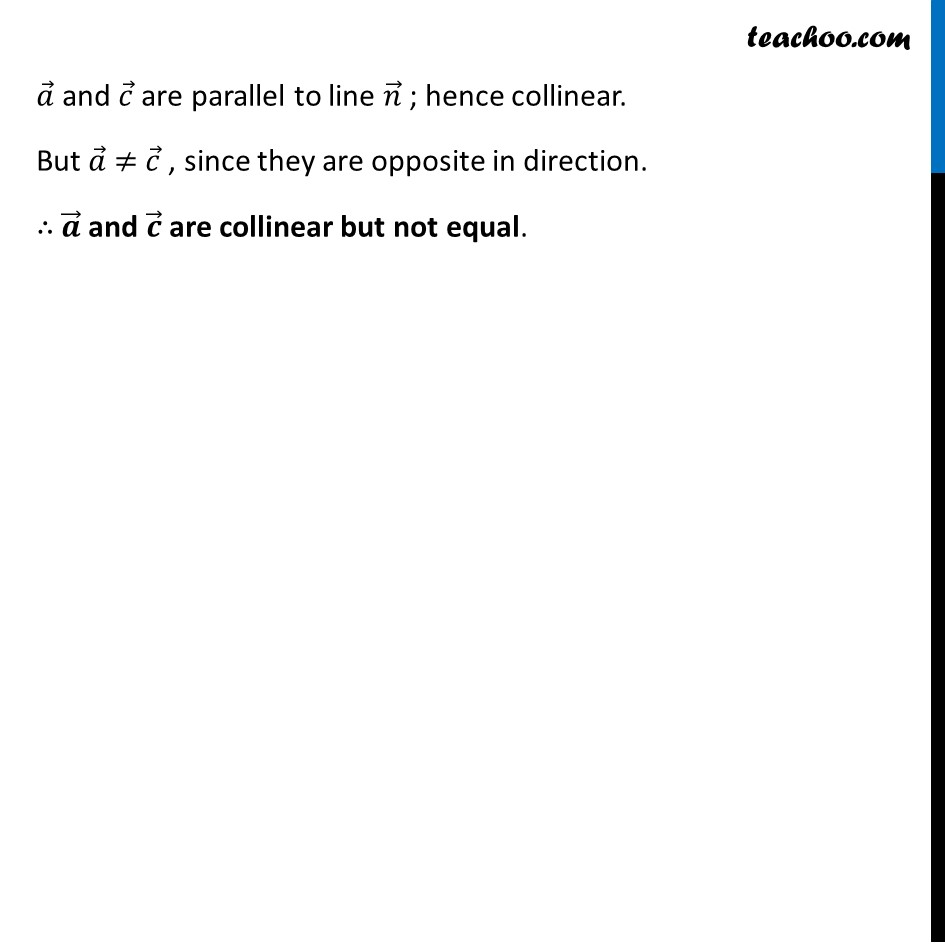Ex 10.1

Chapter 10 Class 12 Vector Algebra
Serial order wiseLearn in your speed, with individual attention - Teachoo Maths 1-on-1 Class

### Transcript

Ex 10.1, 4 In Fig 10.6 (a square), identify the following vectors. (i) coinitialTwo or more vectors are said to be cointial if they start from the same point. Here, 𝑎 ⃗ and 𝑑 ⃗ start from the same point P. So, 𝒂 ⃗ and 𝒅 ⃗ are cointial. Ex 10.1, 4 In Fig 10.6 (a square), identify the following vectors. (ii) EqualTwo or more vectors are said to be equal if they have the same magnitude same direction. Since the given figure is a square, magnitudes of 𝑎 ⃗ , 𝑏 ⃗ , 𝑐 ⃗ and 𝑑 ⃗ are equal. And direction of 𝑏 ⃗ is same as direction of 𝑑 ⃗ Thus, 𝒃 ⃗ and 𝒅 ⃗ are equal. Ex 10.1, 4 In Fig (a square), identify the following vectors. (iii) Collinear but not equalTwo of more vectors are said to be collinear if they are parallel to the same line. 𝑏 ⃗ and 𝑑 ⃗ are parallel to line 𝑚 ⃗ ; hence collinear. But 𝑏 ⃗ = 𝑑 ⃗ 𝑎 ⃗ and 𝑐 ⃗ are parallel to line 𝑛 ⃗ ; hence collinear. But 𝑎 ⃗ ≠ 𝑐 ⃗ , since they are opposite in direction. ∴ 𝒂 ⃗ and 𝒄 ⃗ are collinear but not equal.